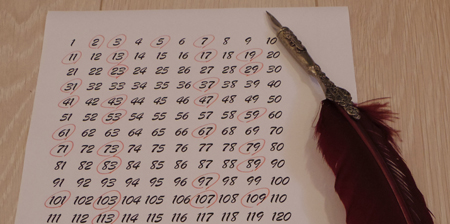CHINEN Koji
Associate Professor Ph D (Mathematical Sciences)

Coding theory is about ways to properly relay information. It is a comprehensive theory that is the result of numerous mathematical developments. Research is conducted with a focus on algebra, including coding theory as a mathematical theory and related group theory, and ring theory. Research is also conducted into analytic number theory as it relates to ciphers.How are the prime numbers distributed ?

Research Area Analytic Number Theory, Coding Theory Dostribution of primitive roots and residual orders, Zeta functions of codes and invariant polynomials (1) Chinen, K. : Extremal invariant polynomials not satisfying the Riemann hypothesis, Appl. Algebra Engrg. Comm. Comput. 30-4 (2019), 275-284.(2) Chinen, K. : Divisible formal weight enumerators and extremal polynomials not satisfying the Riemann hypothesis, Discrete Math. 342 (2019), Article 111601.(3) Chinen, K. : On some families of certain divisible polynomials and their zeta functions, to appear in Tokyo J. Math.

### Applicable Algebra Laboratory

E-mail chinen（at）math.kindai.ac.jp Note that this e-mail address has replaced the "@" with "(at)" to prevent spam. When e-mailing, replace the "(at)" with "@".